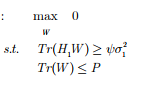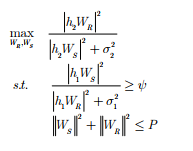# Maximizing SINR

Hi,
This is Saleem,

I am using CVX to maximize the SINR of convex optimization problem, the code is showing error, since i tried many times to debug errors. Anyone here can help me?

My designed problem is:CVX code is:

clear,clc %Clear all
P=4;
m=2;
I=eye(m,m)
G= rand(1,m);
cvx_begin
variable W(m,1);

maximize 0;
subject to
trace(G*W) >=0
trace(W) <= P;
cvx_end

Thanks

The argument of trace must be square. That requirements applies to MATLAB expressions (i.e., numerical arguments) and to CVX expressions… `W` is 2 by 1, so `trace(W)` produces an error message,

I suggest you re-read whatever paper you found this in, and think through what input data makes sense.

Also, note that it is perfectly fine to include the statement
`maximize 0;`
in your CVX code. However, it does nothing, and can be omitted. `maximize 0` (or any constant) , or `minimize 0` (or any constant) has the same effect as not having a maximize statement or minimize statement, namely, to specify a feasibility problem, which is solved if any feasible solution is returned by the solver.This is the actual problem, including two unknown vectors, we can not solve it directly, so i assume one is zero, that’s why max 0;

So according to you a trace will be applied only on square matrix, so instead trace if i am using norm(G*W), it also show errors.

When `G` is 1 by m, as you have then `G*W` is a scalar, so you can take the norm or trace of that (although if `G` were n by m, with n > 1, it might make a more interesting example). Also, norm (in any norm) can be applied to an argument of any dimension.

If you are getting an error, you need to show a complete reproducible example, with all inputs, and show the complete output, including error messages. You might benefit from brushing up on MATLAB basics before trying to use CVX.

You are exactly right. By considering two unknown variables can i solve this problem in CVX?